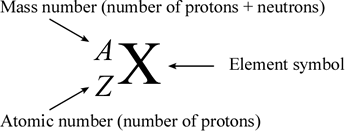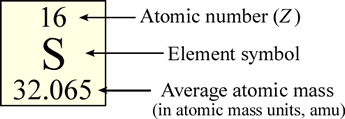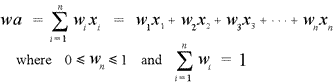CHEM 101
General Chemistry

J. D. Cronk    Syllabus    Topics

GENERAL CHEMISTRY TOPICS

### Isotopes

An isotope is made up of atoms of the same element that have the same atomic mass. Different isotopes of an element arise from atoms with differing numbers of neutrons. Uses of isotopes. Average atomic masses from natural abundances: The weighted-average calculation.

#### Atomic number, mass number and isotopes

Musical accompaniment for these lecture notes.The atomic number of an element (symbolized as Z) is the number of protons in the nuclei of its atoms. The mass number (A) is the total number of nucleons (neutrons and protons). An isotope is made up of atoms of the same element (which by definition have a characteristic and fixed atomic number) that also have the same mass number. Different isotopes of an element arise from atoms with differing numbers of neutrons. Because of this, chemists need a way to represent specific isotopes of an element. Isotopes of an element have the same atomic number, but different mass numbers. The atomic number, when represented along with the symbol of an element, is shown as a leading subscript. The mass number is shown as a leading superscript. Since the element symbol implies an atomic number, the latter is often dropped, and an isotope as commonly represented textually with just the mass number and the element symbol (for example 14C or 18O).In the periodic table, the elements, represented as their symbols, are arranged in a particular pattern that reflects (as we will see) a regularity, or periodicity in their properties. Typically in the table, the element symbol is contained within its own small box, along with other information including the atomic number and the average atomic mass. The average atomic mass of an element represents the averages of its naturally occurring isotopic masses weighted according to their natural abundance. The formula for calculation of average atomic mass and illustration of its use is presented below.

How do the isotopic forms of an element differ from one another, physically and chemically? Isotopes are defined by their subatomic particle composition, which we will think of as a physical property. The chemistry of an element is determined by, in a general sense, the number of valence electrons its atoms possess. The number of valence electrons associated with a neutral atom is in turn determined by the number protons in the nucleus. Thus, two atomic nuclei could have the same number of protons, but different numbers of neutrons. Yet since the atoms they are part of would still have the same number of valence electrons, these two atoms would be chemically indistinguishable.*

#### Uses of isotopes

There are a wide variety of applications of isotopes in nuclear chemistry, medicine, biochemistry, anthropology, paleontology, and geology. Many such uses are based on the phenomenon of radioactivity, shown by some of the isotopes of many of the elements. Such radioactive isotopes are unstable, undergoing spontaneous nuclear decay processes at a rate determined by the half-life of the isotope. One example is the use of 14C - the isotope of carbon with six protons and eight neutrons, which has a half-life of 5730 years - as a basis for dating of materials derived from living organisms that are many thousands of years old. This technique, called radiocarbon dating, is used widely in geosciences and anthropology.

#### Average atomic masses from natural abundances: The weighted-average calculation

The atomic masses given in the periodic table represent weighted averages based on the natural abundances of the isotopes of a given element. The formula for a weighted average isHere the xi's are the masses of the individual isotopes, and the wi's are the fractional abundances corresponding to the isotopes. Note that the weights must sum to 1 (equivalently the percent abundances must sum to 100%).

For example, chlorine exists in two isotopic forms, 35Cl and 37Cl. The mass of the 35Cl isotope is 34.97 amu and that of 37Cl is 36.97 amu. The abundances are 75.77% and 24.23%, repectively. Therefore in this case, the weighted average becomes

wa  =  (0.7577)(34.97 amu)  +  (0.2423)(36.97 amu)  =  35.45 amu

The result of this calculation is the atomic mass of chlorine that appears in the periodic table.

* Actually, since isotopes of an element differ in atomic mass, they can be subtly distinguished by differences in reaction rates, or in physical processes - such as rate of diffusion - affected by mass.